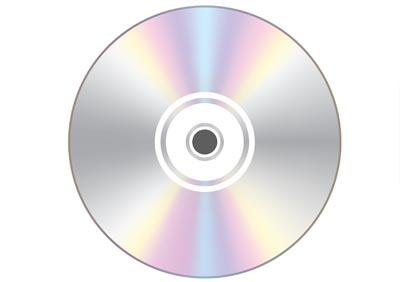# Digital Details [Required for Microcontroller programming]## Digital Basics

Earlier in the digital discussion, I talked about sampling, quantization, and coding. Here, I will explain the details. Digital is simply the expression of information in the form of a code of discrete values.

To create a digital signal from an analog signal, a numerical value is sampled on a time axis through an analog-to-digital (AD) conv

To create a digital signal from an analog signal, a numerical value is sampled (sampling) on a time axis through an analog-to-digital (AD) conversion, and the magnitude of the signal (signal amplitude) is chopped into discrete numerical values, which is called quantization.
Converting the numerical values into binary 0s and 1s is called encoding.

## Sampling

Taking values sporadically in the time direction is called sampling. Sampling is also called sampling period T when expressed in time, and sampling frequency f when expressed in frequency.
The sampling period T is the reciprocal of the frequency T=1/f

For example, the sampling frequency f of an audio CD is 44.1 kHz, so the sampling period T is T=1/(44.1x1000) = 22.676 us.
Incidentally, when the sampling frequency is f, digital signals can be expressed up to half that frequency, f/2. (For details, please check the sampling theorem).

The upper limit of frequencies that normal humans are said to be able to perceive is approximately 20 kHz, so it can be said that the sound quality of a CD satisfies most of this range.

## Quantization

The division of the amplitude direction into several steps is called quantization, and the amount of division is called the quantization bit. The larger the quantization bits, or the higher the resolution of the quantization bits, the finer the amplitude of the signal can be expressed.

A 2-bit quantization bit has 4 steps (2 squared), a 4-bit bit has 16 steps (2 squared), and an n-bit has 2n steps. For example, an audio CD has 16 quantization bits, so there are 65,536 (216) steps.
If the AD converter (analog-to-digital conversion) of a microcontroller has 8 quantization bits (256 steps), for example, the resolution is 5/256 = 0.0195V since it is decomposed by 256 quantization bits in a microcontroller with a maximum analog signal value of 5V.

When image data is captured by image scanners, etc., it is often captured in 8-bit 256 steps of each color (RGB), which is called 24-bit color (8 bits x 3 colors) and can express about 16.77 million steps of colors.
However, since 256 steps of 8 bits per color (RGB) are not very expressive for monochromatic colors, there are also those that support 4,096 steps of 12 bits per color or 16 bits per color.

As the sampling frequency increases and the quantization bits become larger, the signal will approach that of a continuum more closely.